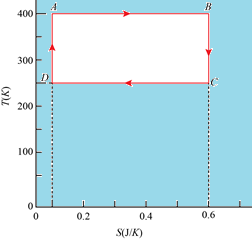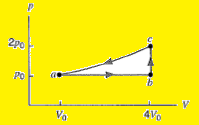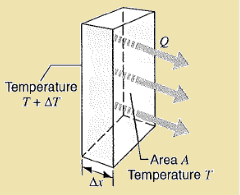Click to Chat

1800-1023-196

+91-120-4616500

CART 0

• 0

MY CART (5)

Use Coupon: CART20 and get 20% off on all online Study Material

ITEM
DETAILS
MRP
DISCOUNT
FINAL PRICE
Total Price: Rs.

There are no items in this cart.
Continue Shopping• Complete Physics Course - Class 11
• OFFERED PRICE: Rs. 2,968
• View Details

```Miscellaneous Exercises Thermal Physics:-

Problem:Icebergs in the North Atlantic present hazards to shipping as shown in the below figure, causing the length of shipping routes to increase by about 30% during the iceberg season. Strategies for destroying icebergs include planting explosives, bombing, torpedoing, shelling, ramming, and painting with lampblack. Suppose that direct melting of the iceberg , by placing heat sources in the ice, is tried. How much heat is required to melt 10% of a 210,000 – metric-ton iceberg? (One metric ton = 1000 kg.)Concept:-

The amount of heat per unit mass that must be transferred to produce a phase change is called the latent L for the process. The total heat transferred in a phase change is then

Q = Lm,

where m is the mass of the sample that changes phase. The heat transferred during melting or freezing is called the heat of fusion.

Solution:

To obtain the heat that is required to melt 10% of a 210,000-metric-ton iceberg, substitute  210,000-metric-ton for mass of the iceberg m and 333 ×103 J/kg for latent heat of fusion of water in the equation Q = 10% (Lm),

Q = 10% (Lm)

= 0.10 (333 ×103 J/kg) (210,000-metric-ton)

= 0.10 (333 ×103 J/kg) (210,000-metric-ton) (103 kg/1 metric-ton)

= 0.10(333 ×103 J/kg) (2.1×108 kg)

= 7.0×1012 J

From the above observation we conclude that, the heat that required to melt 10% of a 210,000-metric-ton iceberg would be 7.0×1012 J.

___________________________________________________________________________

Problem:

For the Carnot cycle shown in below figure, calculate (a) the heat that enters and (b) the work done on the system.Concept:-

In an isothermal process, the heat Q transfer takes place at constant temperature T. The entropy ΔS of the system is defined as,

ΔS = Q/T

Here Q is the positive heat flow into the system. So the entropy ΔS of the system will increase.

From the equation ΔS = Q/T, the absorbed heat Q will be,

Q = (ΔS) (T)

= T (Sf – Si)

Here Sf is the final entropy of the system and Si is the initial entropy of he system.

For a cyclic path,

Q + W = 0

Here Q is the heat added to the system and W is the work done.

Solution:

(a) We have to calculate the heat Qin that enters into the system.

In the above cycle, the heat only enters along the top path AB.

To obtain the heat Qin that enters into the system, substitute 400 K for T, 0.6 J/K for Sf and 0.1 J/K for Si in the equation Q = T (Sf – Si),

Qin = T (Sf – Si)

= (400 K) (0.6 J/K - 0.1 J/K)

= (400 K) (0.5 J/K)

= 200 J

Therefore the heat Qin that enters into the system would be 200 J.

(b) To find the work done, first we have to find out the heat that leaves Qout from the bottom path of the cycle.

In the above cycle, the heat only leaves along the bottom path CD.

Qout = T (Sf – Si)

= (250 K) (0.1 J/K - 0.6 J/K)

= (250 K) (-0.5 J/K)

= -125 J

Thus the heat that leaves Qout from the bottom path of the cycle would be -125 J.

As, Q + W = 0 for a cyclic path, so work done W will be,

W = -Q

= - (Qin+ Qout)        (Since, Q = Qin+ Qout)

To obtain the work done W on the system, substitute 200 J for Qin and -125 J for Qout in the equation W = - (Qin+ Qout),

W = - (Qin+ Qout)

= - (200 J+(-125 J) )

= -75 J

From the above observation we conclude that, the work done W on the system would be -75 J.

______________________________________________________________________________

Problem:

A Carnot engine works between temperatures T1 and T2. It derives a Carnot refrigerator that works between two different temperatures T3 and T4 as shown in the below figure. Find the ratio |Q3| / |Q1| in terms of the four temperatures.Concept:-

The efficiency ε of a Carnot engine is equal to the,

ε = 1- TL/TH

= |W|/|QH|

Here, TL is the temperature of sink (lower temperature), TH is the temperature of source, W is the work done by the engine and QH is the heat at higher temperature.

The coefficient of performance K of the Carnot refrigerator will be,

K = |QL|/W

= TL/TH-TL

Here TL is lower temperature, TH is the higher temperature, W is the work done by the engine and QL is the heat at lower temperature.

Solution:

To obtain the efficiency ε of the Carnot engine, substitute T2 for TL, T1 for TH and Q1 for QH in the equation ε = 1- TL/TH =|W|/|QH|,

ε =1-T2/T1

= |W|/|Q1|

To obtain the coefficient of performance K of the Carnot refrigerator, substitute T4 for TL, T3 for TH and Q4 for QL in the equation K = TL/TH-TL= |QL|/W,

K =T4/T3-T4

= |Q4|/|W|

Lastly, |Q4| = |Q3| - |W|.

We just need to combine these three expressions into one.

From ε =1-T2/T1 = |W|/|Q1|, we get,

1-T2/T1 = |W|/|Q1|

|W| = |Q1| T1-T2/T1

From K =T4/T3-T4 =|Q4|/|W|, we get,

T4/T3-T4 = |Q4|/|W|

= |Q3| - |W|/|W|     (Since, |Q4| = |Q3| - |W|)

= |Q3|/|W| - 1

Substitute the value of |Q1| T1-T2/T1 for W in the equation T4/T3-T4 =|Q3|/|W| - 1, we get,

T4/T3-T4 =|Q3|/|W| - 1

=|Q3|/|Q1| (T1/T1-T2)- 1

So, |Q3|/|Q1| = (T4/T3-T4 +1) (T1-T2/T1)

= (T3/T3-T4) (T1-T2/T1)

= (1- T2/T1) / (1- T4/T3)

From the above observation we conclude that, the ratio|Q3|/|Q1| in terms of the four temperatures would be equal to (1- T2/T1) / (1- T4/T3).

______________________________________________________________________________________

Problem:

One mole of an ideal monoatomic gas is caused to go through the cycle as shown in below figure. Hw much work is done on the gas in expanding the gas from a to c along path abc? (b) What is the change in internal energy and entropy in going from b to c? (c) What is the change in internal energy and entropy in going through one complete cycle? Express all answers in terms of the pressure p0 and volume V0 at a point a in the diagram.Concept:-

Work done W at constant pressure is defined as,

W = pΔV

Here p is the pressure and ΔV is the change in volume.

Change in internal energy ΔE is defined as,

ΔE = 3/2 nRΔT

Here n is the number of moles, R is the gas constant and ΔT is the change in temperature.

Change in entropy ΔS is defined as,

ΔS = 3/2 ln (Tf/Ti)

Here, Tf is the final temperature and Ti is the initial temperature.

Solution:

(a) To obtain the work done Wabc along the path abc, first we have to find out the work done Wab along the path ab.

From the figure we observed that,

Wab = pΔV

= p0 (V0-4V0)

= -3 p0V0

So,   Wabc= Wab

= -3 p0V0

From the above observation we conclude that, the work done on the gas along the path abc would be -3 p0V0.

(b) The change in internal energy ΔEbc along the path b to c would be,

ΔEbc = 3/2 nRΔTbc

= 3/2 (nRTc – nRTb)

= 3/2(pcVc – pbVb)         (pV = nRT)

= 3/2[(2p0) (4V0) -(p0) (4V0)]

= 3/2 [8 p0V0 - 4 p0V0]

= 6 p0V0

The change in entropy ΔSbc along the path b to c would be,

ΔSbc = 3/2nR ln(Tc/Tb)

= 3/2nR ln(Pc/Pb)       (Since, Tc/Tb = Pc/Pb)

=3/2nR ln(2P0/P0)

= 3/2 nR ln2

From the above observation we conclude that, the change in internal energy ΔEbc in the path b to c would be 6 p0V0 and the change in entropy ΔSbc in the path b to c would be 3/2 nR ln2.

(c) The change in entropy and internal energy in a cyclic process is zero. Since the process is a complete cycle, therefore the change in entropy and internal energy in going through one complete cycle would be zero.

_______________________________________________________________________________________________

Problem:

(a) Calculate the rate of heat loss through a glass window of area 1.4 m2 and thickness 3.0 mm if the outside temperature is -20ºF and the inside temperature is +72ºF. (b) A storm window is installed having the same thickness of glass but with an air gap of 7.5 cm between the two windows. What will be the corresponding rate of heat loss presuming that conduction is the only important heat-loss mechanism?

Concept:-

The rate H at which heat is transferred through the rod is,

(a) directly proportional to the cross-sectional area (A) available.

(b) inversely proportional to the length of the rod Δx.

(c) directly proportional to the temperature difference ΔT.

So, H = kA ΔT/ Δx

Here k is the proportionality constant and is called thermal conductivity of the rod.

Again the rate of heat transfer H is defined as,

H = A(T2-T1)/∑Rn

= A ΔT/ (Rg+ Ra)

Here A is the area,  ΔT  is the temperature difference,  Rg is the thermal resistance of glass at the thickness 3.0 mm and Ra is the thermal resistance of air at the thickness 7.5 cm.

The thermal resistance or R-value, defined by,

R = L/k

Here L is the thickness of the material through which the heat is transferred and k is the thermal conductivity of the material.

Solution:

(a) First we have to find the temperature difference between inside and outside of the glass window.

If T2 is the inside temperature and T1 is the outside temperature, then the temperature difference ΔT in °C will be,

ΔT = T2-T1

= 5°C /9 °F (72°F –(-20 ° F))

= 51.1  °C

= (51.1+273) K

= 324.1 K

To obtain the rate of heat loss through a glass window, substitute 1.0 W/m.K (thermal conductivity of window glass) for k, 1.4 m2 for A, 324.1 K for ΔT and 3.0 mm for Δx in the equation H = kA ΔT/ Δx, we get,

H = kA ΔT/ Δx

= (1.0 W/m.K) (1.4 m2) (324.1 K)/ (3.0 mm)

= (1.0 W/m.K) (1.4 m2) (324.1 K)/ (3.0 mm×10-3 m/1 mm)

= 2.4×104 W

From the above observation we conclude that, the rate of heat loss through a glass window would be 2.4×104 W.

(b) First we have to find out the thermal resistance Rg of galss at the thickness 3.0 mm and Ra of air at the thickness 7.5 cm.

To obtain the thermal resistance Rg of galss at the thickness 3.0 mm, substitute 3.0 mm for L and 1.0 W/m.K (thermal conductivity of window glass) for k in the equation R = L/k,

Rg = L/k

= 3.0 mm/1.0 W/m.K

= (3.0 mm×10-3 m/1 mm) /(1.0 W/m.K)

= 3.0×10-3 m2.K/W

To obtain the thermal resistance Ra of air at the thickness 7.5 cm, substitute 7.5 cm for L and 0.026 W/m.K (thermal conductivity of dry air) for k in the equation R = L/k,

Ra = L/k

= 7.5 cm /0.026 W/m.K

= (7.5 cm ×10-2 m/1 mm) /(0.026 W/m.K)

= 2.88 m2.K/W

To find out the corresponding rate of heat loss H, substitute 1.4 m2 for A,3.0×10-3 m2.K/W for Rg and 2.88 m2.K/W for Ra in the equation H = A ΔT/ (Rg+ Ra), we get,

H = A ΔT/ (Rg+ Ra)

= (1.4 m2) (324.1 K) /(3.0×10-3 m2.K/W) (2.88 m2.K/W)

= 25 W

From the above observation we conclude that, the corresponding rate of heat loss H would be 25 W.

___________________________________________________________________________________________________

Problem:

Consider the slab as shown in the below figure. Suppose that Δx = 24.9 cm, A = 1.80 m2, and the material is copper. If T = -12.0ºC, ΔT = 136ºC, and a steady state is reached, find (a) the temperature gradient, (b) the rate of heat transfer, and (c) the temperature at a point in the rod 11.0 cm from the high temperature end.Concept:-

The temperature gradient is defined as,

Here ΔT is the temperature difference and Δx is the thickness of the slab.

The rate H at which heat is transferred through the slab is,

(a) directly proportional to the area (A) available.

(b) inversely proportional to the thickness of the slab Δx.

(c)  directly proportional to the temperature difference ΔT.

So, H = kA ΔT/ Δx

Here k is the proportionality constant and is called thermal conductivity of the material.

Solution:

(a) To obtain the temperature gradient, substitute 136 ° C for ΔT and 0.249 m for Δx in the equation, temperature gradient = ΔT/Δx,

= (136 ° C)/(24.9 cm)

= (136 +273) K/( 24.9 cm×10-2 m/1 cm)

= 409 K /0.249 m

= 1643 K/m

Therefore the temperature gradient of the slab will be 1643 K/m.

(b) To find out the rate of heat transfer H of the slab, substitute 1.80 m2 for the area A, 401 W/m. K for the thermal conductivity k of copper and1643 K/m for ΔT/Δx in the equation H = kA ΔT/ Δx,

H = kA ΔT/ Δx

= (401 W/m. K) (1.80 m2 ) (1643 K/m)

= 11.85×105 W

Therefore the rate of heat transfer H of the slab will be 11.85×105 W.

(c) ΔT = TH – T

Here T is the temperature at a point in the rod 11.0 cm from the high temperature end.

So, TH = ΔT + T

To find out TH, substitute 136 ° C for ΔT and -12 ° C for T in the equation TH = ΔT + T,

TH = ΔT + T

= (136 +273) K + (-12+ 273 )

= 409 K + 261 K

= 670 K

We know that temperature gradient is,

ΔT/Δx = 1643 K/m

(TH – T)/Δx = 1643 K/m     (Since, ΔT = TH – T)

TH – T = (1643 K/m) Δx

T = TH - (1643 K/m) Δx

To find out T, substitute 670 K for TH and 11.0 cm for Δx in the equation T = TH - (1643 K/m) Δx,

T = TH - (1643 K/m) Δx

= (670 K) – ((1643 K/m) (11.0 cm))

= (670 K) – ((1643 K/m) (11.0 cm))

= (670 K) – ((1643 K/m) (11.0 cm×10-2 m/1 cm ))

= (670 K) – ((1643 K/m) (0.11 m)

= (670 K) – (180.73 K)

= 489.27 K

Rounding off to two significant figures, the temperature at a point in the rod 11.0 cm from the high temperature end will be 490 K.

Related Resources:-

You might like to thermodynamics.

For getting an idea of the type of questions asked, refer the  Previous Year Question Papers.

To read more, Buy study materials of Thermodynamics comprising study notes, revision notes, video lectures, previous year solved questions etc. Also browse for more study materials on Chemistry here.

```### Course Features

• 101 Video Lectures
• Revision Notes
• Previous Year Papers
• Mind Map
• Study Planner
• NCERT Solutions
• Discussion Forum
• Test paper with Video Solution